# Filtering repeating values in excel join datatable

Hi team,

I am getting below table -

join.xlsx (10.2 KB)

I want to remove lines

• when a cost unit occurs in a subsequent series then take from the series the last
• when a cost unit line doesnt have direct following row with the same cost unit, then block it
• I only want to select one row if a unit cost repeats.
For example lines 3,5,7,9,11.

Is there any way to do it ?

Regards,
G

Case is about detecting duplicates based on 1 column and deduplication by taking the last group member. When UnitCost occurs only 1 time, then also keeps it?

Assign Activity:
dtFiltered =

``````(From d in dtData.AsEnumerable
Group d by k=d("UnitCost").toString.ToUpper.Trim into grp=Group
Select g = grp.Last()).CopyToDataTable
``````

When case is as described above but unique UnitCosts rows are also to get blocked, then we can do:
Assign Activity:
dtFiltered =

``````(From d In dtData.AsEnumerable
Group d By k=d("UnitCost").toString.ToUpper.Trim Into grp=Group
Where grp.Count > 1
Select g = grp.Last()).CopyToDataTable
``````

When the first group members are to keep the we just use first() instead of last()

``Select g = grp.First()).CopyToDataTable``
1 Like

Hi @gokul1904
Use following syntax in assign activity and assign this to datatable:
In Assign stage:

Blockquote →
outputDT = inputDT.AsEnumerable.GroupBy(function(r) r(“UnitCost”)).Where(function(r) r.Count() > 1).SelectMany(function(l) l).ToArray().CopyToDatatable

I am getting below error while trying to assign it -

@gokul1904 I think so Its linq code you should put invoke code Activity.

1 Like

Yeah unique unitcosts rows also get blocked.So can we use first() and last() to filter in the same datatable?

last() only gives the last filtered unique unit costs and first() gives the first ones.

Is there a way to use both together?

when

then

``````(From d In dtData.AsEnumerable
Group d By k=d("UnitCost").toString.ToUpper.Trim Into grp=Group
Where grp.Count > 1
Select g = grp.Last()).CopyToDataTable
``````

So it will group the data on CostUnit
we will have Duplicates when the group member count > 1
so we filter for only these groups
and take from the group last member

For example lines 3,5,7,9,11.

1 Like

By using this -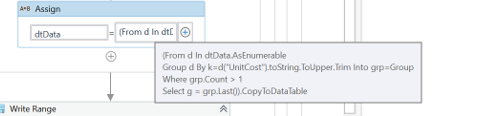I only got lines 7,9,11.

So how do I get 3 and 5 ?

Regards,

show the output from immediate panel when typing in dtData befoe applying the LINQ, so we can inspect the origin datatable content

Before Linq -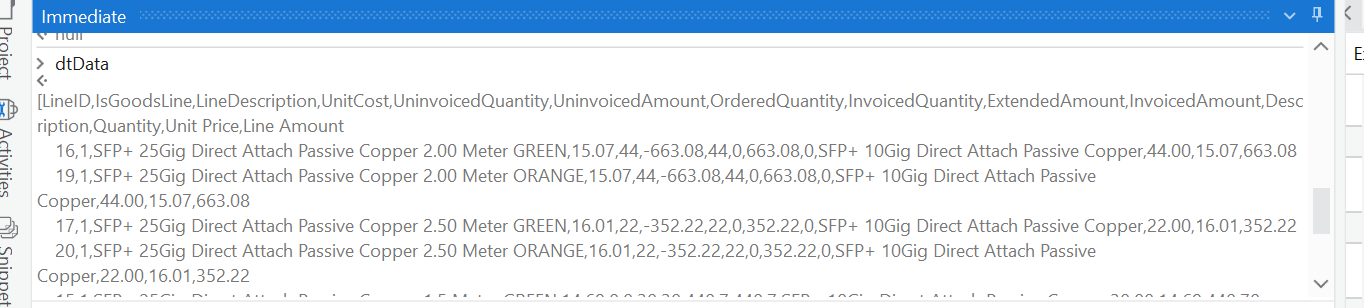After Linq -

here is the confusion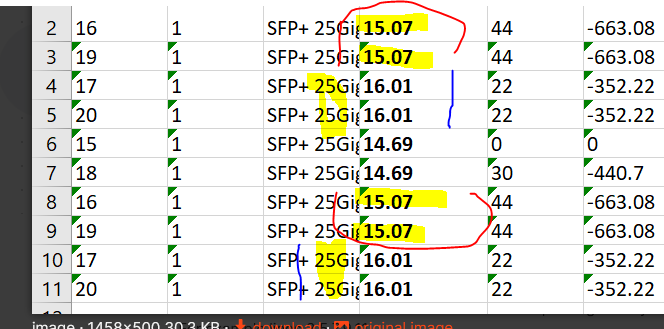as a duplicated group eg 15.07 has 4 or maybe more members

So we would recommend to rewrite the requirements from

to

• when a cost unit occurs in a subsequent series then take from the series the last
• when a cost unit line doesnt have direct following row with the same cost unit, then block it

just check the requirement and when it is ok, then we renter the solution option check phase

Apologies for the confusion -

I change my problem statement to the below -

• when a cost unit occurs in a subsequent series then take from the series the last
• when a cost unit line doesnt have direct following row with the same cost unit, then block it

Also updated in the main post

Regards,

1 Like

Hi @ppr did you find any Linq query to achieve the same?

Regards,

@gokul1904
we do feel that following approach is matching better to the case as it less blackbox and can better be inspected e.g. during debugging

Variables:

Flow - Segmenting:

``````valCheck = dtData.AsEnumerable.Skip(mySkip).First()("Col1").toString.ToUpper.Trim
ExtractSegments = ExtractSegments.Append(dtData.AsEnumerable.Skip(mySkip).TakeWhile(Function (x) x("Col1").toString.ToUpper.Trim.Equals(valCheck)).toList).ToList
mySkip = ExtractSegments.SelectMany(Function (x) x).Count
``````

in a do while

• retrieve the check value
• skip or start by 0
• extract the subsequent Rows, where the Col1 value is equal to the check Value
• Calculate the new Skip

Flow - Deduplication & Result Datatatable Building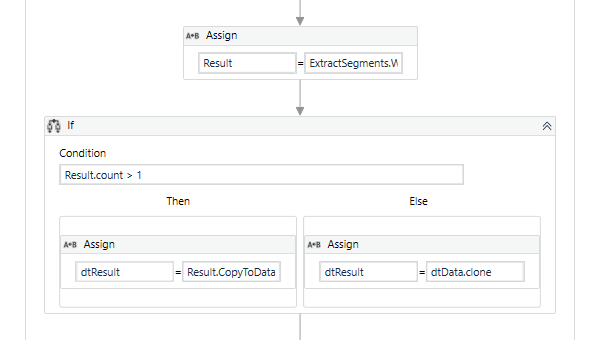``````Result = ExtractSegments.Where(Function (x) x.Count > 1).Select(Function (x) x.Last()).toList
``````

Sample Date (testing also the no duplicate case)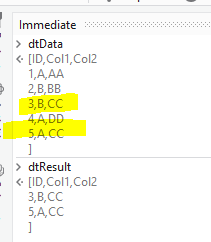Part result: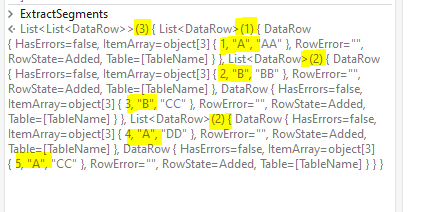Find starter help here:
TakeWhile_MakeSeriesGroup_TakeLastFromDuplicate.xaml (12.2 KB)

1 Like

Thank you @ppr
but at present when I am processing the attached file
join - Copy.xlsx (9.8 KB)

it is not returning any rows whereas expected was to skip the 2nd duplicate row

Please let me know if I am missing anything. Also it should return the same datatable incase there is no match - for below files -

join.xlsx (9.8 KB)
join_01.xlsx (9.8 KB)

Regards,
g

just handle outcomes seperated:

• A: Protoype code in its box where do see the results or fails
• B: the adapted own implementation

For A: show the case of fail by get bound to the sample data schema
For B: share with us your implementation

where data is:

with a given requirement / discussions and mentioning:

where we also see:

then it is explained, why a single line is blocked. Looks like a defect in the requirement definition.

but with the highlight to filter give a try at:

``````Result = ExtractSegments.Select(Function (x) x.Last()).toList
``````
1 Like

for this feedback we also would recommmend to reread the above communications and refer to the explanation of First() and Last.

However we encourage you to play with the things (also one reason why we focused the approach supporting debugging and tracing) as you explore and change as you do need it.

This worked thanks -
Result = ExtractSegments.Select(Function (x) x.Last()).toList

This topic was automatically closed 3 days after the last reply. New replies are no longer allowed.